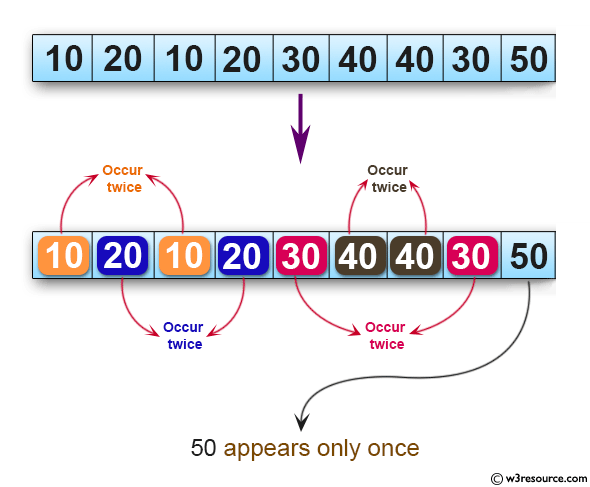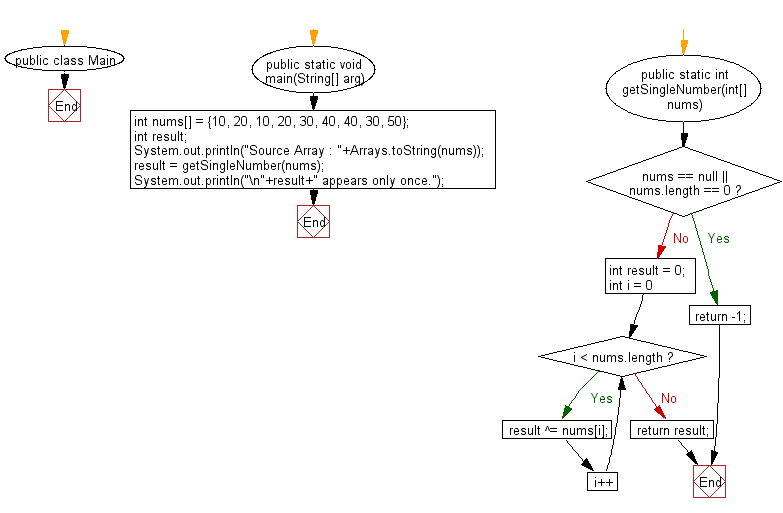﻿ Java: Find a number that appears only once in a given array# Java Exercises: Find a number that appears only once in a given array of integers, all numbers occur twice

## Java Basic: Exercise-129 with Solution

Write a Java program to find a number that appears only once in a given array of integers, all numbers occur twice.
Example: {10,2,38,23,38,23,21}
Output: 23

Pictorial Presentation:Sample Solution:

Java Code:

``````import java.util.*;
public class Main {
public static  void main(String[] arg)
{
int nums[] = {10, 20, 10, 20, 30, 40, 40, 30, 50};
int result;
System.out.println("Source Array : "+Arrays.toString(nums));
result = getSingleNumber(nums);
System.out.println("\n"+result+" appears only once.");
}

public static int getSingleNumber(int[] nums) {
if(nums == null || nums.length == 0) {
return -1;
}
int result = 0;
for (int i = 0; i < nums.length; i++) {
result ^= nums[i];
}
return result;
}
}
```
```

Sample Output:

```Source Array : [10, 20, 10, 20, 30, 40, 40, 30, 50]

50 appears only once
```

Flowchart:Java Code Editor:

What is the difficulty level of this exercise?

Test your Programming skills with w3resource's quiz.

﻿

## Java: Tips of the Day

getEnumMap

Converts to enum to Map where key is the name and value is Enum itself.

```public static <E extends Enum<E>> Map<String, E> getEnumMap(final Class<E> enumClass) {
return Arrays.stream(enumClass.getEnumConstants())
.collect(Collectors.toMap(Enum::name, Function.identity()));
}
```

Ref: https://bit.ly/3xXcFZt